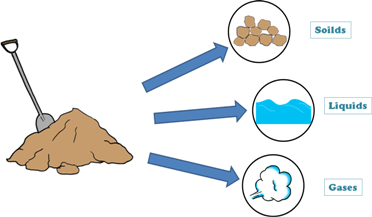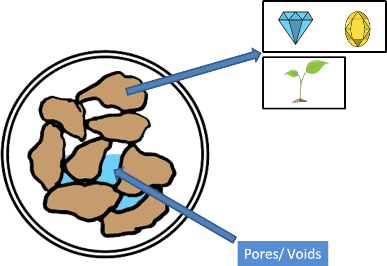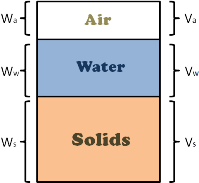Soil Phase DiagramA soil mass in general is composed of three kinds of matter,

1. Solids

2. Liquid

3. Gaseous

Solids can be minerals or organic matters or both. These solids can be of different sizes and shapes and because of it they enclose empty spaces. These empty spaces are called pores or voids.These voids in the soil mass are either filled by liquid, which is generally water, or by gases, which is usually air, or they are filled by both, partly by water and partly by air. If voids are not occupied by the water then they are occupied by the air.

The proportions of solids, water and air in a soil mass influence its physical properties. And before founding any structure on the soil we should study the soil in detail including its physical properties.

We can imagine a soil mass with its constituents (i.e. solids, water and air) separated, though these different constituents present in the soil mass cannot be separated, but it will be helpful in understanding the soil behavior and deriving its properties.We can think of constituents occupying separate spaces. Volume of solids in the soil mass is marked as Vs, volume of water is as Vw and volume of air as Va.

The diagrammatic representation of different phases seperated in soil mass is called phase diagram.

A partially saturated soil, which voids are partially filled with water and air, contains three phases viz solid, water and air. Hence it is called 3 phase Diagram.

When all the voids are completely filled with water, the soil becomes saturated and the gaseous phase becomes absent from the diagram. Now the phase diagram will contain only two phases, solids and water, so it is called 2 phase Diagram.

Similarly when the soil is completely dry, all the soil pores are empty or we can say filled with air, again a 2 phase diagram is received.

Now from the 3 phase diagram we can notice – the total volume of soil sample capital V, is equal to the sum of volume of solids Vs plus volume of water Vw plus volume of air Va.

V = Vs + Vw + Va

Also the total weight of the soil sample W is equal to the weight of solids Ws plus weight of water Ww plus weight of air Wa.

But for all practical purposes weight of air is considered as zero, hence weight of soil sample is only weight of solids Ws plus weight of water Ww.

W = Ws + Ww

One important thing to note here is that the term voids is used to include both the filled and the unfilled proportions of the pore spaces enclosed by the soil grains.

Tags : phase system

Published on :2019-08-04